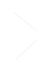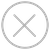TensorSpace.js
Getting StartBasic ConceptsTensorSpace ConverterModel PreprocessingModelsLayersMerge FunctionUsage Example
This section introduces the usage of TensorSpace-Converter for different types of pre-trained model from TensorFlow, Keras, TensorFlow.js.TensorFlow
A pre-trained model built by TensorFlow can be saved as saved model, frozen model, combined HDF5 model or separated HDF5 model. Use different TensorSpace-Converter commands for different kinds of TensorFlow model formats. TensorSpace-Converter collects the data from tensor, then use the outputs as the inputs of layer of TensorSpace visualization. The developer can collect all necessary tensor names and set the name list as output_layer_names.
tf.keras model
For a combined HDF5 model, topology and weights are saved in a combined HDF5 file xxx.h5. Set input_model_format to be tf_keras. The sample command script should be like:
``````\$ tensorspacejs_converter \
--input_model_from="tensorflow" \
--input_model_format="tf_keras" \
--output_layer_names="layer1Name,layer2Name,layer3Name" \
./PATH/TO/MODEL/xxx.h5 \
./PATH/TO/SAVE/DIR``````
tf.keras (separated)
For a separated HDF5 model, topology and weights are saved in separate files, topology file xxx.json and weights file xxx.h5. Set input_model_format to be tf_keras_separated. In this case, the model have two input files, merge two file's paths and separate them with comma (.json first, .h5 last), and then set the combined path to positional argument input_path. The sample command script should be like:
``````\$ tensorspacejs_converter \
--input_model_from="tensorflow" \
--input_model_format="tf_keras_separated" \
--output_layer_names="layer1Name,layer2Name,layer3Name" \
./PATH/TO/MODEL/xxx.json,./PATH/TO/MODEL/eee.h5 \
./PATH/TO/SAVE/DIR``````
frozen model
For a TensorFlow frozen model. Set input_model_format to be tf_frozen. The sample command script should be like:
``````\$ tensorspacejs_converter \
--input_model_from="tensorflow" \
--input_model_format="tf_frozen" \
--output_layer_names="layer1Name,layer2Name,layer3Name" \
./PATH/TO/MODEL/xxx.pb \
./PATH/TO/SAVE/DIR``````
saved model
For a TensorFlow saved model. Set input_model_format to be tf_saved. The sample command script should be like:
``````\$ tensorspacejs_converter \
--input_model_from="tensorflow" \
--input_model_format="tf_saved" \
--output_layer_names="layer1Name,layer2Name,layer3Name" \
./PATH/TO/SAVED/MODEL/FOLDER \
./PATH/TO/SAVE/DIR``````
Checkout this TensorFlow Preprocessing Tutorial for more practical usage of TensorSpace-Converter for TensorFlow models.Keras
A pre-trained model built by Keras, may have two formats: topology and weights are saved in a single HDF5 file, or topology and weights are saved in separated files. Use different TensorSpace-Converter commands for these two saved Keras models.
Combined .h5
For a Keras model, topology and weights are saved in a single HDF5 file, i.e. xxx.h5. Set input_model_format to be topology_weights_combined. The sample command script should be like:
``````\$ tensorspacejs_converter \
--input_model_from="keras" \
--input_model_format="topology_weights_combined" \
--output_layer_names="layer1Name,layer2Name,layer3Name" \
./PATH/TO/MODEL/xxx.h5 \
./PATH/TO/SAVE/DIR``````
Separated .json & .h5
For a Keras model, topology and weights are saved in separated files, i.e. a topology file xxx.json and a weights file xxx.h5. Set input_model_format to be topology_weights_separated. In this case, the model have two input files, merge two file's paths and separate them with comma (.json first, .h5 last), and then set the combined path to positional argument input_path. The sample command script should be like:
``````\$ tensorspacejs_converter \
--input_model_from="keras" \
--input_model_format="topology_weights_separated" \
--output_layer_names="layer1Name,layer2Name,layer3Name" \
./PATH/TO/MODEL/xxx.json,./PATH/TO/MODEL/eee.h5 \
./PATH/TO/SAVE/DIR``````
Checkout this Keras Preprocessing Tutorial for more practical usage of TensorSpace-Converter for Keras models.TensorFlow.js
A pre-trained model built by TensorFlow.js, may have a topology file xxx.json and a weights file xxx.weight.bin. To converter the model with TensorSpace-Converter, the two files should be put in the same folder and set topology file's path to input_path. The sample command script should be like:
``````\$ tensorspacejs_converter \
--input_model_from="tfjs" \
--output_layer_names="layer1Name,layer2Name,layer3Name" \
./PATH/TO/MODEL/xxx.json \
./PATH/TO/SAVE/DIR``````
Checkout this TensorFlow.js Preprocessing tutorial for more practical usage of TensorSpace-Converter for TensorFlow.js models.